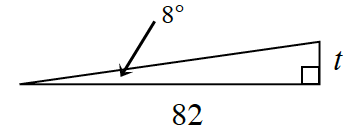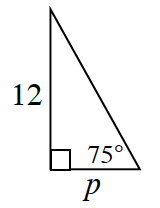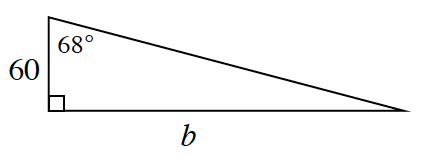### Home > INT2 > Chapter 3 > Lesson 3.2.4 > Problem3-105

3-105.

Calculate the missing side length for each triangle. Use the tangent button on your calculator to help.

1.$\text{tan}(8^\circ) =\frac{\Delta y}{\Delta x} = \frac{\textit{t}}{82}$

$82(\tan8^\circ)=t$

$t ≈ 11.524$

1.$p ≈ 3.215$

1.Rotate the triangle so that $68^\circ$ is the slope angle, then use the same method as in parts (a) and (b).